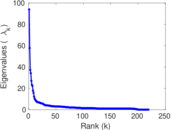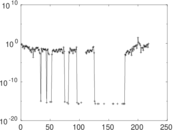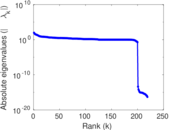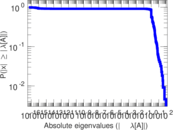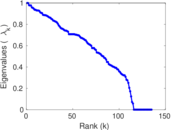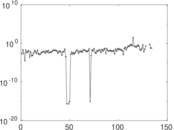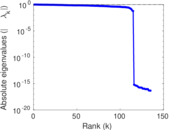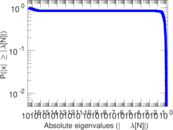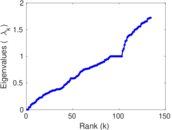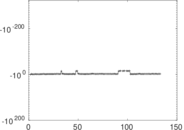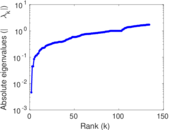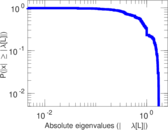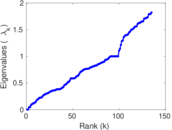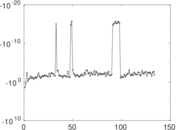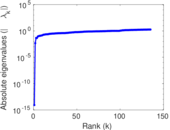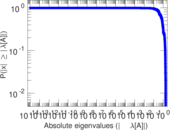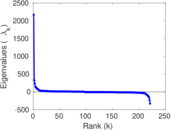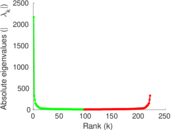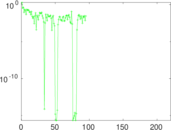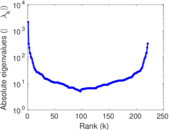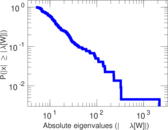# Wikiquote edits (ur)

This is the bipartite edit network of the Urdu Wikiquote. It contains users and pages from the Urdu Wikiquote, connected by edit events. Each edge represents an edit. The dataset includes the timestamp of each edit.

 Code `qur` Internal name `edit-urwikiquote` Name Wikiquote edits (ur) Data source http://dumps.wikimedia.org/ AvailabilityDataset is available for download Consistency checkDataset passed all tests Category Authorship network Dataset timestamp 2017-10-20 Node meaning User, article Edge meaning Edit Network formatBipartite, undirected Edge typeUnweighted, multiple edges Temporal dataEdges are annotated with timestamps

## Statistics

 Size n = 2,169 Left size n1 = 221 Right size n2 = 1,948 Volume m = 3,995 Unique edge count m̿ = 2,455 Wedge count s = 836,069 Claw count z = 335,377,861 Cross count x = 104,934,267,562 Square count q = 6,530 4-Tour count T4 = 3,401,650 Maximum degree dmax = 2,121 Maximum left degree d1max = 2,121 Maximum right degree d2max = 90 Average degree d = 3.683 73 Average left degree d1 = 18.076 9 Average right degree d2 = 2.050 82 Fill p = 0.005 702 57 Average edge multiplicity m̃ = 1.627 29 Size of LCC N = 1,932 Diameter δ = 15 50-Percentile effective diameter δ0.5 = 3.127 21 90-Percentile effective diameter δ0.9 = 7.678 45 Median distance δM = 4 Mean distance δm = 4.120 16 Gini coefficient G = 0.688 368 Balanced inequality ratio P = 0.227 159 Left balanced inequality ratio P1 = 0.120 401 Right balanced inequality ratio P2 = 0.325 156 Relative edge distribution entropy Her = 0.745 336 Power law exponent γ = 5.781 56 Tail power law exponent γt = 2.781 00 Tail power law exponent with p γ3 = 2.781 00 p-value p = 0.000 00 Left tail power law exponent with p γ3,1 = 1.941 00 Left p-value p1 = 0.546 000 Right tail power law exponent with p γ3,2 = 3.121 00 Right p-value p2 = 0.000 00 Degree assortativity ρ = −0.263 893 Degree assortativity p-value pρ = 2.157 92 × 10−40 Spectral norm α = 93.935 2 Algebraic connectivity a = 0.004 614 54 Spectral separation |λ1[A] / λ2[A]| = 1.628 09 Controllability C = 1,760 Relative controllability Cr = 0.814 815

## Plots

### Fruchterman–Reingold graph drawing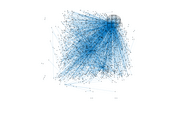### Degree distribution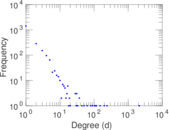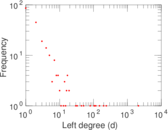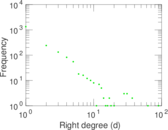### Cumulative degree distribution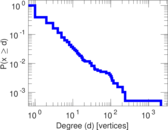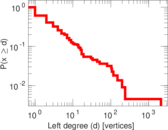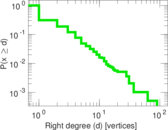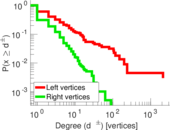### Lorenz curve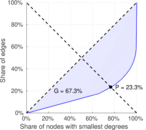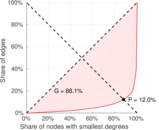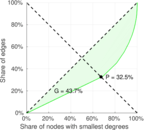### Spectral distribution of the adjacency matrix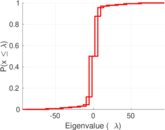### Spectral distribution of the normalized adjacency matrix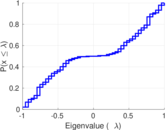### Spectral distribution of the Laplacian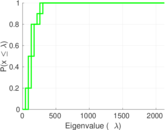### Spectral graph drawing based on the adjacency matrix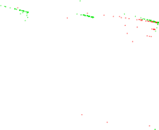### Spectral graph drawing based on the Laplacian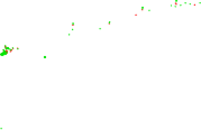### Spectral graph drawing based on the normalized adjacency matrix### Degree assortativity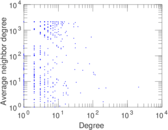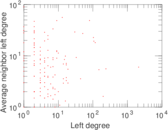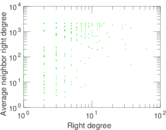### Zipf plot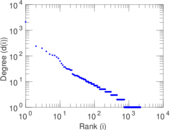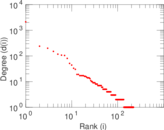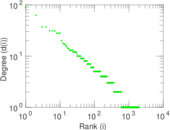### Hop distribution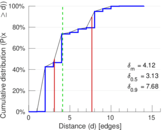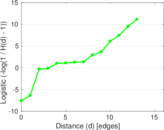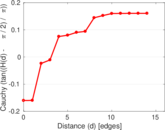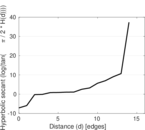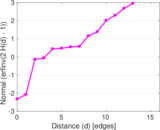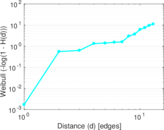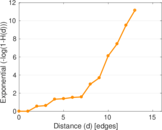### Double Laplacian graph drawing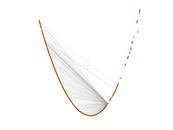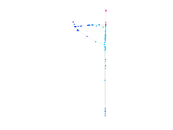### Delaunay graph drawing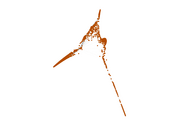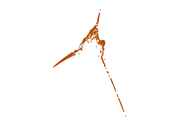### Edge weight/multiplicity distribution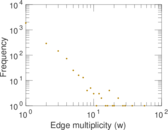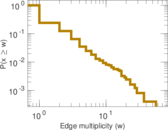### Temporal distribution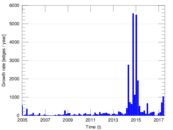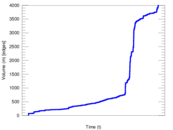### Temporal hop distribution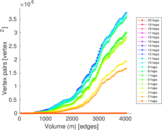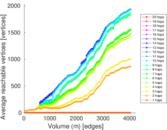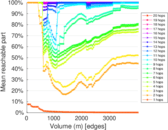### Diameter/density evolution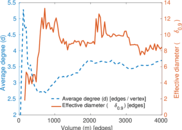### Matrix decompositions plots